### Home > CAAC > Chapter 2 > Lesson 2.1.2 > Problem2-18

2-18.
1. Remember that one meaning of the word “evaluate” is to replace a variable with a number and to calculate the result. For example, when asked to evaluate the expression x2 when x = −2, the solution would be (−2)2 = 4.
Evaluate the expressions below for the given values. Homework Help ✎

1. −4d + 3 if d = −1

2. km if k = 4 and m = −10

3.if t = 6 and w = −3

4. x 2 + y 2 if x = 7 and y = 5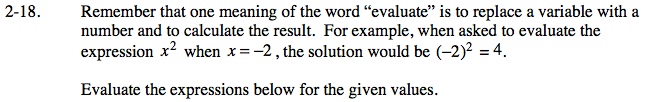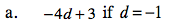Substitute −1 for d.

−4(−1) + 3

Simplify the terms and combine them.

4 + 3 = 7

7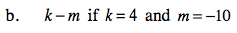Follow the steps in part (a).

Put everything you substituted inside parenthesesso you do not lose your negative signs.

(4) − (−10)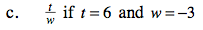Follow the steps in part (a).

−2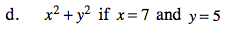Follow the steps in part (a).